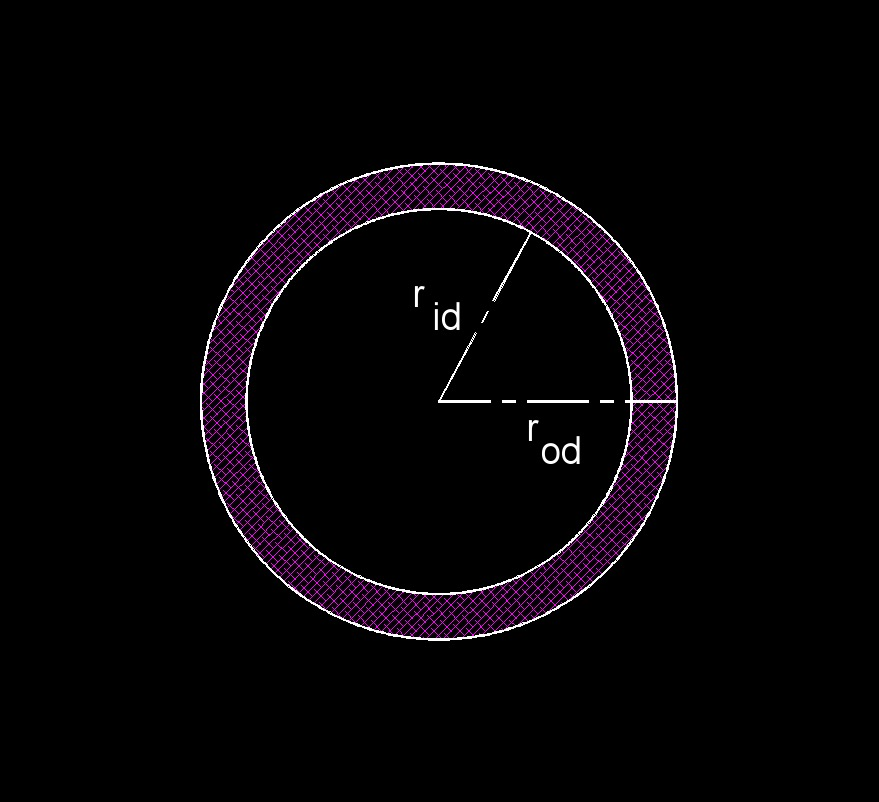# Annulus

Written by Jerry Ratzlaff on . Posted in Plane GeometryThe area between two concentric circles.

### Annulus formula

$$\large{ A= \pi \;r_{id}^2 - \pi \; r_{od}^2 }$$

$$\large{ A= \pi \; \left( r_{id}^2 - r_{od}^2 \right) }$$

Where:

$$\large{ A }$$ = area

$$\large{ \pi }$$ = Pi

$$\large{ r_{id} }$$ = radius (inside diamester)

$$\large{ r_{od} }$$ = radius (outside diameter)